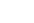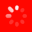The No.1 Website for Pro Audio
Search This Thread  Search This Forum  Search Reviews  Search Gear Database  Search Gear for sale  Search Gearslutz Go Advanced
Gear Maniac

Passive Sum two signals - Resistor Value Help

Hello folks,

I am putting together a pedal board for my two acoustic guitars which each have a pair of pickups in them.

Basically I want to sum each pair to mono and have been doing some reading on the values keeping in mind impedances of the chain.

The two signals to sum would be coming from two separate preamp/EQs with output impedances of 1Kohm and 100 ohms respectively.

After summing they would be going into a Hall of Fame Reverb which has an input impedance of 1 meg Ohm, if bypassed I guess the summing network would "see" the DI box placed after the Reverb which also has input impedance of 1Mohm.

Going by the 10:1 rule that would dictate that I need 10Kohm resistors to sum the two channels prior to the TC Reverb pedal given that one of the EQs has an output impedance of 1K.
I understand that 10Kohm resistors would give a 10Kinput impedance at the summing network and roughly a 6db drop in signal.

Is 10K the best value to use or is there a gremlin in these details that I am missing?

Perhaps it would be best to sum after the reverb given that the reverb pedal has a lower output impedance (100 ohms) and accepts a stereo signal. Bypassed of course though I would be in the same scenario.

Any help or advice greatly appreciated!!

Lives for gear

Assuming the preamp output levels are similar, put a 900 ohm resistor in series with the low impedance output and Y it together with the high impedance output. You can probably fit the resistor inside a connector shell when you build the Y cable.

David L. Rick
Seventh String Recording

PS: For a quicker answer in the future, post questions like this to the Geekslutz forum.
Gear Maniac

Quote:
Assuming the preamp output levels are similar, put a 900 ohm resistor in series with the low impedance output and Y it together with the high impedance output. You can probably fit the resistor inside a connector shell when you build the Y cable.

David L. Rick
Seventh String Recording

PS: For a quicker answer in the future, post questions like this to the Geekslutz forum.
Thank you for your help. Noted regarding the geekslutz section.

Thanks again
Lives for gear

why would you have a 6dB drop?
Never heard of a 10:1 rule for summing resistors..
Gear Maniac

Quote:
why would you have a 6dB drop?
Never heard of a 10:1 rule for summing resistors..

The 10:1 was the general simplification that I have seen mentioned time and again with regards to the output and input impedances. One of my outputs is 1K which led me to thinking I would need a summing network with at least 10K input impedance.

http://www.epanorama.net/circuits/linesum.html
That's where I got the 6db loss and 10K input impedance figures from.

Attenuation: 6 dB
Input impedance: 10 kohm
Output impedance: 5 kohm

I guess these figures in isolation don't mean anything and change depending on what is hooked up either side? As you can tell I am working my way through this and trying to learn a little along the way.

I received the following explanation elsewhere explaining that the summing resistors alone do not set the input impedance.

"Ignoring the 1Mohm of the Receiver, the output of one Source sees the output of the other Source as it's input.
say you have the 100ohm output feeding into the 10k and then into another 10k feeding into the 1k output impedance of the other Source.
The 100ohm outputs sees 10k+10k+1k as it's load.
The 1kohm output sees 10k+10k+100 as it's load.
There is a small reduction in load when the 1Mohm is paralleled into the loading at the 10k junction.
Both Sources will be able to drive those loads."

So with a pair of 10K summing resistors summing my two signals, the input impedance is above 20Kohm going by the above.

How would I calculate the loss due to the summing in the above example with a pair of 10K resistors summing two signals (100ohms and 1Kohms output impedance)?

Thanks.

Last edited by woodnut; 18th October 2016 at 02:31 PM.. Reason: extra detail
Gear Maniac

*If* I have understood the theory correctly the minimum signal loss of summing two signals passively will be 6db but the actual result depends on the impedance of the input following the summing network.

Am I correct in my understanding that the summing circuit can be seen as a simple voltage divider where the input is the summing resistor at 10K and the value of the shunt is the input impedance of the next stage (D.I. Box 1Meg Ohm) in parallel with the other 10K summing resistor (9.9K).

9.9K/(9.9K + 10K) = 0.4975 is this just over a 6 db drop?
Topic:

Welcome to the Gearslutz Pro Audio Community!

Registration benefits include:
• The ability to reply to and create new discussions
• Interact with VIP industry experts in our guest Q&As
• Get INSTANT ACCESS to the world's best private pro audio Classifieds for only USD \$20/year
• Remove this message!
You need an account to post a reply. Create a username and password below and an account will be created and your post entered.